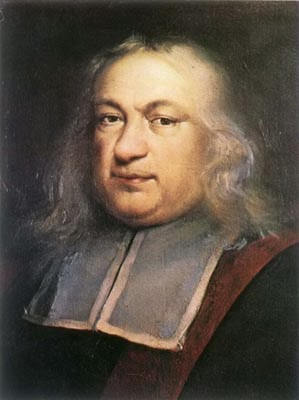## Saturday, 13 September 2014

### Fermat's ConjectureProf. Wiles, in reprose
Fermat was a brilliant French mathematician of the 17th century. He was also a mischievous individual who liked to tease his contemporaries with mathematical conundrums. Fermat delighted in the frustration and impotence experienced by lesser minds especially when they failed to solve his equations.

Unlike science, mathematics can provide absolute knowledge. Once a theorem has been proved, that proof will stand for all time and is not subject to change or modification. Contrast this with scientific knowledge. A scientific theory may be subject to revision or be totally replaced by a new theory which better explains a particular phenomenon.

Fermat had the annoying habit of not committing to paper his calculations when it came to producing proofs and solving equations. In the margins of a copy of: 'Arithmetica' he scribbled: "I have a remarkable proof for this equation but have insufficient space to write it here".  He was commenting with regard to a deceptively simple equation, whose general form can be represented thusly:
Xn + Yn= Zn . Where n equals 2 the equation represents Pythagoras's famous theorem about right angled triangles.

However, there is a dark postulate that there are no whole number solutions for the equation where n is greater than 2.  Anyone can do the math but it takes a genius to produce the proof.

I suspect Fermat's solution was flawed, but we will never know. In the 1994, the English mathetician, Andrew Wiles produced a proof which is valid and sound. But he used modern mathematical techniques which would have been unavailable to the 17th century French savant.

Of course this advance in number theory has no relevance to practical knowledge. Prof. Wiles sequestered himself away for 8 years to solve this most arcane of problems, and produced mathematical poetry. Silly sod.Pierre looking cool

#### 1 comment:

1.Try X=1, Y=0, Z=1 and n is any number. Simples...